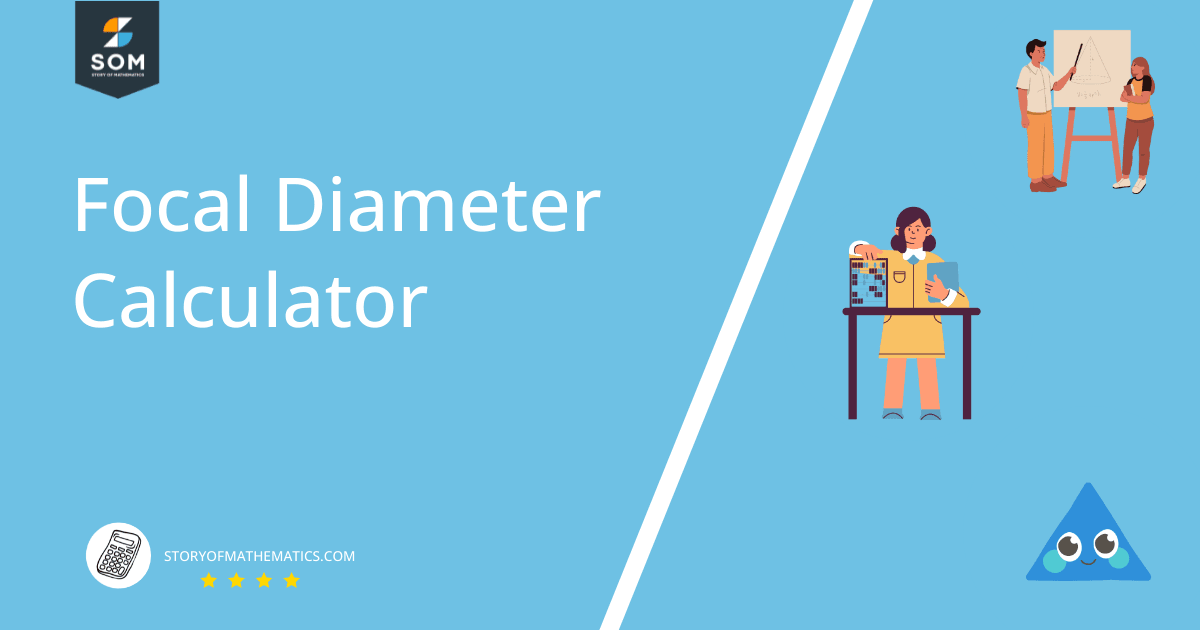# Focal Diameter Calculator + Online Solver With Free Steps

A Focal Diameter Calculator is a calculator used for tracking the line going through the focal point of a parabola which is the point of convergence of the parabola. This line segment is called the Focal Diameter.

The equation is entered into the calculator which then calculates and displays all these properties on the output screen.## What Is a Focal Diameter Calculator?

A Focal Diameter Calculator is an online tool that can be easily used to determine the focal diameter of a Parabola.

It is also used to determine other properties of parabola such as focus, vertex, semi-axis length, directrix, focal parameter, and eccentricity by just inserting the equation into the calculator.

A Focal Diameter Calculator is useful for the detailed solution of questions related to the focal diameter of a parabola. The equation is entered into the calculator with at least two variables and the maximum power of the variable to be 2 as required for a parabola. The calculator provides all the answers on the output window.

## How To Use a Focal Diameter Calculator?

You can start using this calculator by developing an equation for which you need to determine the focal diameter. The following steps should be followed to determine the properties of a parabola using the Parabola Calculator:

### Step 1

Enter the equation into the empty box titled Equation.

### Step 2

Press the Submit button below the input box to view the results.

### Step 3

An output window appears with all the properties of the parabola displayed in a sequence.

### Step 4

You can keep using this calculator to obtain the solution to other problem equations as well.

## How Does a Focal Diameter Calculator Work?

A Focal Diameter Calculator works by determining the longest distance from the focal point to the edge or vertex of the parabola. It is a calculator that can be handy in getting all the properties of the parabola equation entered as input into the calculator.

The following properties of a given parabola can be determined using this calculator:

### Focus

Focus is the point from where all points of the parabola are an equal distance away.

### Vertex

The point where the parabola intersects the axis is called a vertex.

### Semi-axis Length

Semi-axis length is the length of half of the axis.

### Focal Parameter

It is the distance between the focus to the directrix.

### Eccentricity

It is the distance between the focus and any point on the parabola. The eccentricity of a parabola is always 1.

### Directrix

Directrix is the line drawn parallel to the axis at a distance.

## Solved Examples

### Example 1

Consider the following equation:

$x^2-3y+6=0$

Determine the focal diameter, directrix, eccentricity, and vertex of the above parabolic equation.

### Solution

The following properties of the parabola equation are displayed on the output screen:

Focus:

$[0, \dfrac{11}{4}] = (0, 2.75)$

Vertex:

(0,2)

Semi-axis Length:

$\dfrac{3}{4} = 0.75$

Focal Parameter:

$\dfrac{3}{2} = 1.5$

Eccentricity:

Directrix:

$y=\dfrac{5}{4}$

### Example 2

Calculate the focal diameter of the following equation:

$(x-2)^2+y=0$

### Solution

The following results are obtained using calculator for $(x-2)^2+y=0$ parabola:

Focus:

$[2, \dfrac{-1}{4}] = (2, -0.25)$

Vertex:

(2,0)

Semi-axis Length:

$\dfrac{1}{4} = 0.25$

Focal Parameter:

$\dfrac{1}{2} = 0.5$

Eccentricity:

Directrix:

$y=\dfrac{1}{4}$

### Example 3

Consider:

$2y^2-x=3$

Calculate the focal diameter and all the properties of the parabola given above.

### Solution

By putting the parabola $2y^2-x=3$  in the calculator, the following results are obtained:

Focus:

$[\dfrac{-23}{8},0] = (-2.875, 0)$

Vertex:

(-3,0)

Semi-axis Length:

$\dfrac{1}{8} = 0.125$

Focal Parameter:

$\dfrac{1}{4} = 0.25$

Eccentricity:

1

Directrix:

$x=\dfrac{-25}{8}$